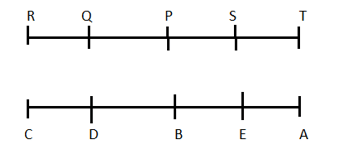# Reasoning Quiz for IBPS CLERK PRE 2019 | 19 November 2019

## Reasoning Quiz for IBPS CLERK PRE

Reasoning Quiz to improve your  Reasoning  for SBI Po & SBI clerk exam Reasoning, IBPS PO Reasoning , IBPS Clerk Reasoning , IBPS RRB Reasoning, LIC AAO , LIC Assistant,  and other competitive exams

Directions (Q1-Q5): Study the following information to answer the given questions:

Ten people are sitting in two parallel rows containing five people each, in such a way that there is an equal distance between adjacent persons. In row 1, P, Q, R, S and T are seated and all of them are facing south. In row 2, A, B, C, D and E are seated and all of them are facing north. Therefore, in the given seating arrangement, each member seated in a row faces another member of the other row.

D sits third to the left of A. P faces immediate neighbor of D. R sits second to the right of P. Only one person sits between Q and S. B and E are immediate neighbors. E does not face P and Q.

Q1.How many persons are seated between Q and T?

(a) None

(b) One

(c) Two

(d) Three

(e) Cannot be determined

Q (1 – 5):Q1. Ans.(c)

P and S

Q2.Four of the following five are alike in a certain way and thus form a group. Which is the one that does not belong to that group?

(a)R

(b) S

(c)C

(d) T

(e)A

Q (1 – 5):Q2. Ans.(b)

All other are sitting at the ends.

Q3. Who among the following are sitting exactly in the middle of the rows?

(a)P,E

(b) S, D

(c) S, A

(d)A, R

(e) P, B

Q (1 – 5):Q3. Ans.(e)

Q4. Which of the following is true regarding B?

(a) A and C are immediate neighbors of B.

(b) B sits at one of the extreme ends of the line.

(c) Q faces B.

(d) T is an immediate neighbor of the person facing B.

(e) D sits on the immediate left of B.

Q (1 – 5):Q4. Ans.(e)

Q5. Four of the following five are alike in a certain way and thus form a group. Which is the one that does not belong to that group?

(a) T— E

(b) Q — C

(c) S — B

(d)R—A

(e) P — D

Q (1 – 5):Q5. Ans.(d)

In all other, the second is the neighbor of the one facing the first.

Direction(6-8):  Study the following information carefully and answer the questions given below:
Six Boys i.e. P, Q, R, S, T and F are of different heights. No two persons are of same height. P is taller than R, S and F. T is taller than only two persons. Q is shorter than P but just taller than R. F is shorter than S. S is shorter than R.
Q8. Who among the following is just taller than T?
1. P
2. R
3. Q
4. S
5. None of these
Q6. Ans(2)Q7. How many persons are taller than S?
1. One
2. Two
3. Three
4. Four
5. More than four
Q7. Ans(4)Q8. Who among the following is the shortest?
1. P
2. R
3. Q
4. F
5. None of these
Q8. Ans(4)Q.9 In a certain code “DONE” is coded as “ WLMV” and “ WINK” is coded as “ DRMP”, then what will be the code for the word “ WALL”?
1. ZOOD
2. ODOZ
3. DOZO
4. DZOO
5. None of these
Q9. Ans(4)Q.10 How many such numerals are there in the number ‘28194302’ which will remain at the Same position when arranged in ascending order from right to left?
1. One
2. Two
3. Three
4. Four
5. None
Q10. Ans(1)### You Can Read This Also:

Best E-books for LIC AAO 2019 : Get PDF here

ambitiousbaba.com need your support to Grow

I challenge you will get Best Content in Our PDFs with Detail solutions and Latest Pattern

Memory Based Puzzle E-book | 2016-19 Exams Covered

Get PDF here

Caselet Data Interpretation 200 Questions

Get PDF here
Puzzle & Seating Arrangement E-Book for BANK PO MAINS (Vol-1)

Get PDF here

### ARITHMETIC DATA INTERPRETATION 2019 E-book

Get PDF here
The Banking Awareness 500 MCQs E-book| Bilingual (Hindi + English)

Get PDF here

High Level DATA INTERPRETATION Practice E-BOOK

Get PDF her